# Representing graphs for neural networks

Note that this is the solutions to the first session, for the work notebook go here

You can find the online version of this notebook here

In this first section we will look at how to go from an simple graph representation to an input suitable for neural networks.

First we’ll look at some basics ways of representing graphs and the differencce between directed and undirected graphs.

Then we’ll go through the adjacency matrix.

In the end we look at graph attributes. We’ll discuss how we will have to handle different kind of variables differently and what this means for encoding the graphs for our neural networks.

Try to answer the sections marked as Question. The sections marked Task has programming task you should perform.

## Representing graphs in code

A graph is generally defined as a pair of sets, a set of nodes and a set of edges between them $$G = (N, E)$$. To be able to tell nodes apart, we need to assign a unique identifier to each node, which we’ll refer to as an index.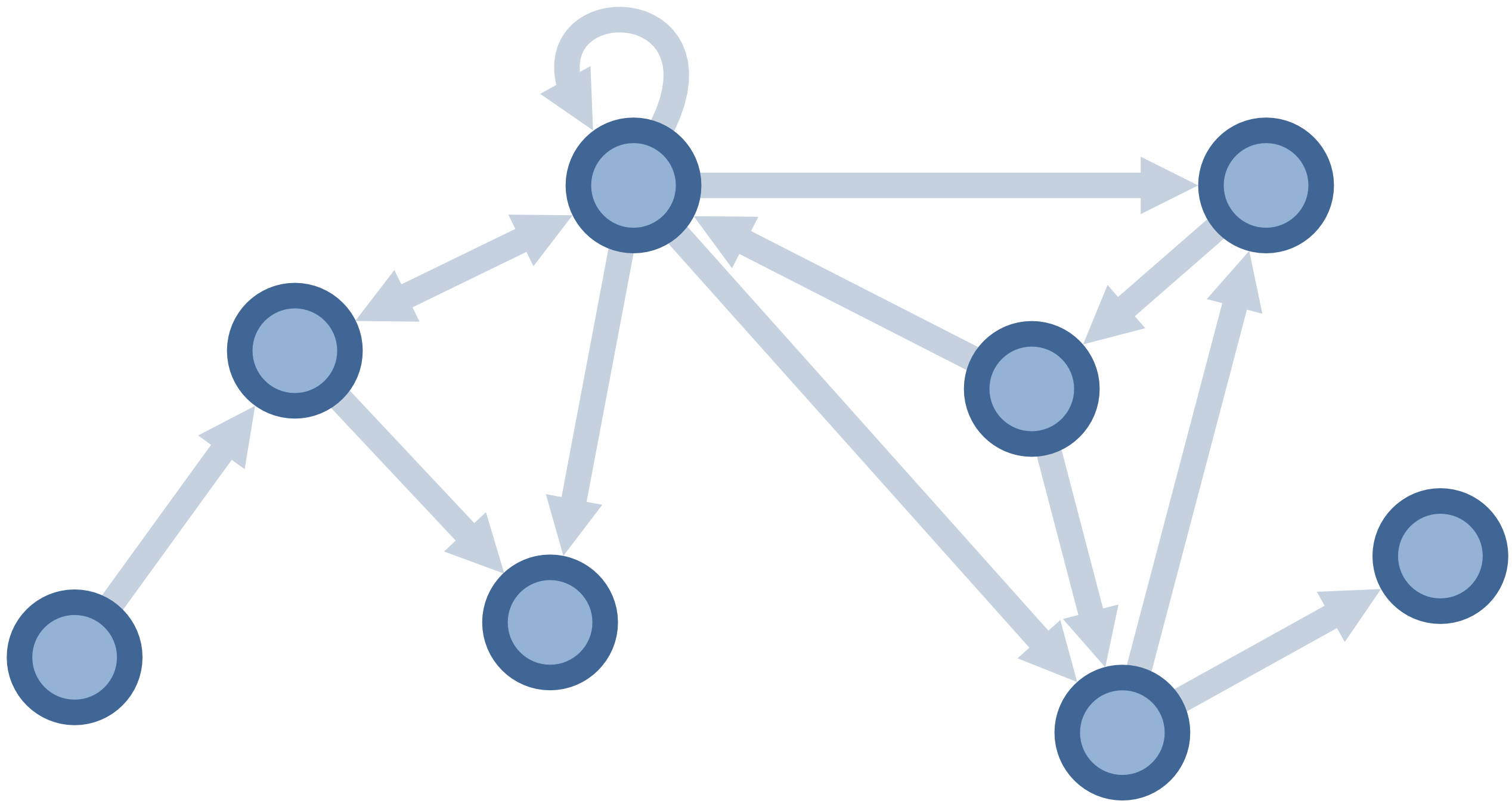Each edge is defined by the nodes it connects, and is therefore represented as a pair (or tuple) of nodes. If the graph is explicitly undirected, the order of the nodes in the pair is not important, but in a directed graph the edge $$(u, v)$$ is different from the edge $$(v, u)$$. A common way of representing graphs is to always assume that it’s a directed graph, and to represent undirected graphs by adding the edge in both directions. While this might seem wasteful, it often allows algorithms to be applicable to both directed and undirected graphs without modification.

A simple representation of a directed graph in python can look as follows:

nodes = {0, 1, 2, 3, 4, 5, 6, 7}
edges = {(0,1), (1,2), (2,1), (1,3), (2,2), (2,3), (2,4), (2,6), (4,5), (5,2), (5,6), (6,4), (6,7)}
graph = (nodes, edges)

print('datatype nodes:', type(nodes), '(',
len(nodes), 'x',
type(min(nodes)), ')')
print('datatype edges:', type(edges), '(',
len(edges), 'x',
type(min(edges)), '(',
type(min(edges)), ',',
type(min(edges)), '))')
print('datatype graphs:', type(graph))

datatype nodes: <class 'set'> ( 8 x <class 'int'> )
datatype edges: <class 'set'> ( 13 x <class 'tuple'> ( <class 'int'> , <class 'int'> ))
datatype graphs: <class 'tuple'>


Question

Why is there a mismatch in number of edges in the code compared to the figure?

### Undirected graph and permutation invariance

In the example above, relationships between nodes was not symmetric, so the relationship $$(1,2)$$ does not imply $$(2,1)$$. A real world example of this directed graph would be e.g a follower graph on a social network.

In many cases, the relationship is symmetric, so $$(1,2)$$ is the same as $$(2,1)$$. We will refer to this property as permutation invariance, changing the order of the nodes in the edge of an undirected graph is still the exact same graph.

### Permutation invariance in python

Below is a figure of an undirected graph followed by python code representing this undirected graph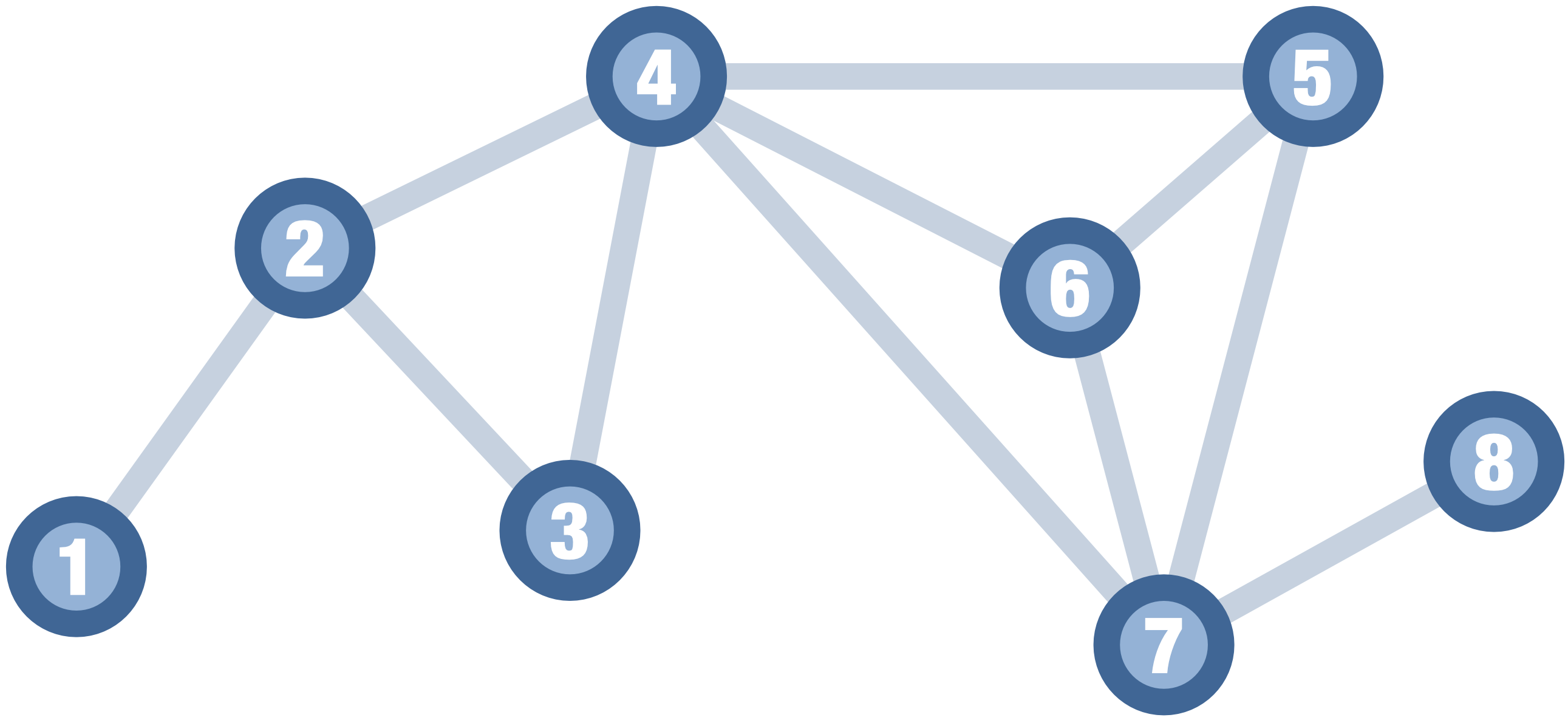def compare_graphs(g1, g2):
nodes_1, edges_1 = g1
nodes_2, edges_2 = g2
return nodes_1 == nodes_2 and edges_1 == edges_2

# Lists
nodes_1 = [1, 2, 3, 4, 5, 6, 7, 8]
edges_1 = [(1,2), (2,3), (2,4), (3,4), (4,5), (4,6), (4,7), (5,6), (5,7), (6,7), (7,8)]
g1 = (nodes_1, edges_1)

nodes_2 = [2, 4, 5, 3, 8, 1, 6, 7]
edges_2 = [(2,1), (2,3), (4,2), (3,4), (5,4), (4,6), (4,7), (6,5), (5,7), (7,6), (7,8)]
g2 = (nodes_2, edges_2)

# Sets
nodes_3 = {1, 2, 3, 4, 5, 6, 7, 8}
edges_3 = [{1,2},  {2,3}, {2,4}, {3,4}, {4,5}, {4,6}, {4,7}, {5,6}, {5,7}, {6,7}, {7,8}]
g3 = (nodes_3, edges_3)

nodes_4 = {2, 4, 5, 3, 8, 1, 6, 7}
edges_4 = [{2,1}, {2,3}, {4,2}, {3,4}, {5,4}, {4,6}, {4,7}, {6,5}, {5,7}, {7,6}, {7,8}]
g4 = (nodes_4, edges_4)

# Print
print("Is g1 and g2 the same graph?", compare_graphs(g1, g2))

print("Is g3 and g4 the same graph?", compare_graphs(g3, g4))

Is g1 and g2 the same graph? False
Is g3 and g4 the same graph? True


Question

Why is the comparison results different for g1 and g2 vs. g3 and g4?

Question

How could you represent an undirected graph using lists or tuples for the edges (i.e. ordered datastructures)?

We’ve represented the graph as a list of edges, but another common representation is that of an adjacency matrix. Since an edge essentially represents a pairwise relationship, we can encode these relationships in a matrix $$A$$ of size $$\text{number of nodes} \times \text{number of nodes}$$, where each element $$A_{i,j}$$ encodes the relationshop between these nodes. When this matrix encodes the edge relationships, we typically set the positions representing an edge to $$1$$ and the positions representing pairs without edges to $$0$$.

The adjecancy matrix will play a central role in our later discussions on Graph Neural Networks and Transformers.

### Adjacency matrix of a directed graph

Each element encode a non-symmetrical relationship, so how to interpret and write the adjacency matrix is ambiguous. Here we will use the convention that the each row $$i$$ encodes the outgoing edges from node $$i$$, converesly each column $$j$$ encodes the incoming edges to node $$j$$.

As discussed above, we can still represent symmetric relationships between nodes by adding edges in both directions.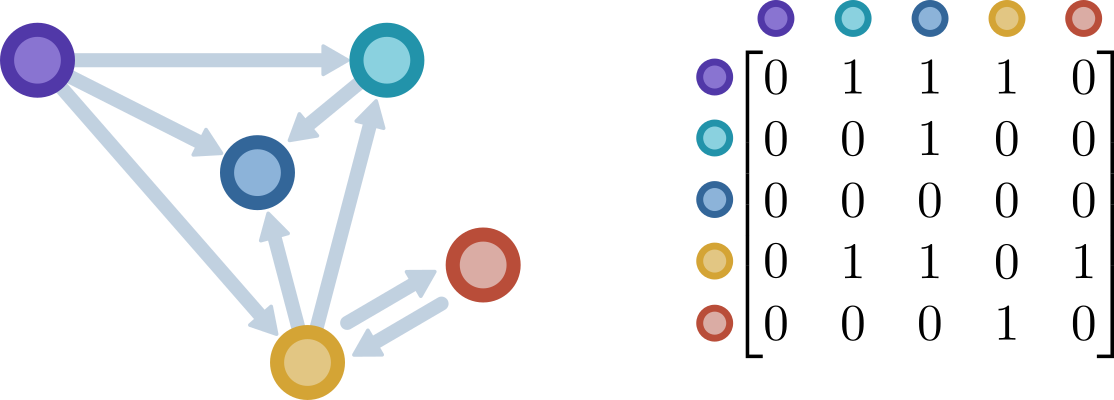### Adjacency matrix of an undirected graph

In an undirected graph, the adjecency matrix is symmetric since it expresses a symmetric relationship between entitites. That is, the following holds:

$A_{ij} = A_{ji}$

In this sense, we can think of an undirected graph as a special case of directed graphs, where the adjacency matrix is symmetric.

By then using the adjacency matrix as the representation for our algorithms (in our Graph Neural Network), we don’t have to implement special cases for undirected graphs, we just treat all graphs as directed and leave it up to the data loading to create the desired adjacency matrix.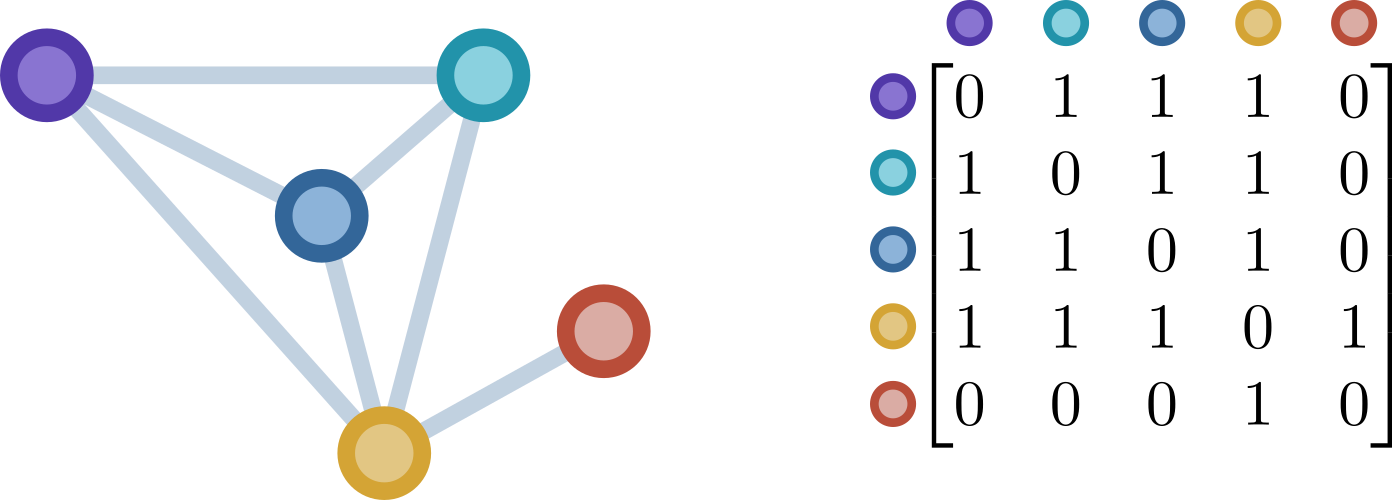Fill in the adjacency matrix for the following graph in the text cell below it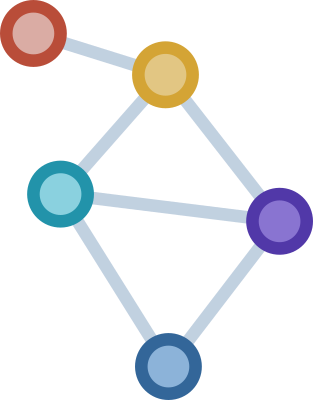Edit this cell to fill in the adjacency matrix

$\begin{split}\begin{bmatrix} 0& ?& ?& ?& ?\\ ?& 0& ?& ?& ?\\ ?& ?& 0& ?& ?\\ ?& ?& ?& 0& ?\\ ?& ?& ?& ?& 0\\ \end{bmatrix}\end{split}$

Fill in the adjacency matrix for the following directed graph in the text cell below it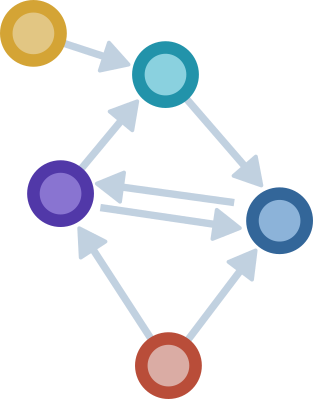Edit this cell to fill in the adjacency matrix

$\begin{split}\begin{bmatrix} 0& ?& ?& ?& ?\\ ?& 0& ?& ?& ?\\ ?& ?& 0& ?& ?\\ ?& ?& ?& 0& ?\\ ?& ?& ?& ?& 0\\ \end{bmatrix}\end{split}$

Question

For each of the graphs above, are their adjacency matrices unique? Why/why not?

Question

What would a non-zero entry in the diagonal of the adjacency matrix encode, and when would we want to use that?

implement the function below which takes a set of edges and produces an adjacency matrix

nodes = {1,2,3,4,5}
edge_set = {(1,2), (2,4), (3,2), (3,4), (4,3), (5,3), (5,4)}

working_matrix = [*len(nodes) for i in range(len(nodes))]
# Can you rely on the node labels corresponding to indices?
# some_code_here
for edge in edges:
u, v = edge
# Set the correct element, can you use *u* and *v* directly?
# some code here
return working_matrix


[[0, 0, 0, 0, 0],
[0, 0, 0, 0, 0],
[0, 0, 0, 0, 0],
[0, 0, 0, 0, 0],
[0, 0, 0, 0, 0]]


## Graph attributes

We’ve looked at how the structure of a graph can be encoded. In particular the adjacency matrix gives us all the information about the pairwise relationships of a graph.

What about other things which could be useful for our graph learning, such as color of nodes or weights of edges?

Consider the structural formula of caffeineThese structural formulas are essentially graphs, but the nodes and edges (atoms and bonds) have attributes associated with them. The nodes (atoms) for example are of different type (e.g. carbon, oxygen, nitrogen) just like the edges (bonds) also are of different type (e.g. single, double or aromatic).

Below is an example of how we might represent the molecule as a graph.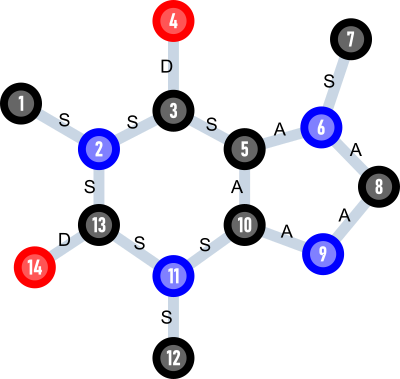Note that atom type is encoded by colour and bond type by a symbol (S-single, D-double, A-aromatic), while each node has been assigned a unique integer index. To represent this in code, we need to keep track of the atom features (atom type) and bond features (node attributes). We can represent this simply using dictionaries.

caffein_nodes = {1: {'atom_type': 'C'},
2: {'atom_type': 'N'},
3: {'atom_type': 'C'},
4: {'atom_type': 'O'},
5: {'atom_type': 'C'},
6: {'atom_type': 'N'},
7: {'atom_type': 'C'},
8: {'atom_type': 'C'},
9: {'atom_type': 'N'},
10: {'atom_type': 'C'},
11: {'atom_type': 'N'},
12: {'atom_type': 'C'},
13: {'atom_type': 'C'},
14: {'atom_type': 'O'}}

# frozenset is an immutable set which can be used as dictionary keys.
# This way caffeine_edges[frozenset({a,b})] == caffeine_edges[frozenset({b,a})]
# so we don't have to care about the order in which we express the nodes of an
# edge
caffeine_edges = {frozenset({1,2}): {'bond_type': 'S'},
frozenset({2,3}): {'bond_type': 'S'},
frozenset({2,13}): {'bond_type': 'S'},
frozenset({3,4}): {'bond_type': 'D'},
frozenset({3,5}): {'bond_type': 'S'},
frozenset({5,6}): {'bond_type': 'A'},
frozenset({5,10}): {'bond_type': 'A'},
frozenset({6,7}): {'bond_type': 'S'},
frozenset({6,8}): {'bond_type': 'A'},
frozenset({8,9}): {'bond_type': 'A'},
frozenset({9,10}): {'bond_type': 'A'},
frozenset({10,11}): {'bond_type': 'S'},
frozenset({11,12}): {'bond_type': 'S'},
frozenset({11,13}): {'bond_type': 'S'},
frozenset({13,14}): {'bond_type': 'D'}}